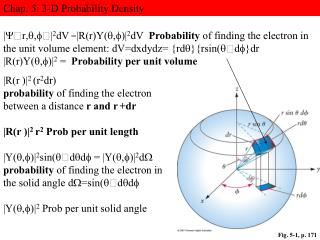DownloadDownload PresentationFig. 5-1, p. 171

# Fig. 5-1, p. 171

Download Presentation## Fig. 5-1, p. 171

- - - - - - - - - - - - - - - - - - - - - - - - - - - E N D - - - - - - - - - - - - - - - - - - - - - - - - - - -
##### Presentation Transcript

1. Chap. 5: 3-D Probability Density r|2dV =|R(r)Y()|2dV Probabilityof finding the electron in the unit volume element: dV=dxdydz= {rd}{rsin(d}dr |R(r)Y()|2 = Probability per unit volume |R(r )|2 (r2dr) probability of finding the electron between a distance r and r+dr |R(r )|2 r2 Prob per unit length |Y()|2sin(dd= |Y()|2d probability of finding the electron in the solid angle d=sin(dd |Y()|2 Prob per unit solid angle Fig. 5-1, p. 171

2. Chap. Solid Angle for a Sphere of Radius r The solid angleddA/r2 = sin(dddV= dr dA(surface area of dV), dA = r2sin(dd A=4πr2(surface area of the sphere)r2 = 4π solid angle portended(projected) by a sphere Solid angle DDA/r2 dA= r2sin(dd Differential Area DW r

3. Chap. Solid Angle for a Sphere of Radius r The solid angleddA/r2 = sin(dddV= dr dA(surface area of dV), dA = r2sin(dd A=4πr2(surface area of the sphere)r2 = 4π solid angle portended(projected) by a sphere Solid angle DDA/r2 DW Looking through D and thusDA to find the electron r

4. =Zr/a0 • Recall that the Bohr radii are rn= n2/Za0 Table 5-2, p. 175

5. Chap 5: Quantum Mechanical Energy levels The Energy Eigen Values are Independent of l and m! Therefore each n level has n, l levels (0,1, ..n-1), each with (2l+1) states and is therefore n2 degenerate. Each (nl) level has (2l+1) m-states and is (2l+ 1) degenerate

6. Chap 5: y1s~R10( r )Y00(, y2s~R20( r )Y00(, y3s~R30( r ) Y00( Number of Nodes in Rnl(r) (n-l-1): radial nodes langular nodes Y00( = 1/√4π a0 the Bohr is most probable radius Sphere Radius rs Prob < 0.05 of max prob for finding electron at r > rs

7. Fig. 5-7, p. 201

8. Contour Map of the LA Basin

9. Contour Map of the LA Basin Topographical Map of the LA Basin

10. Chap 5: Angular Momentum Eigen functions Ypz= Ypz Ypy Ypz=Y10~cos() Linear Combinations of Eigen Functions Ypx=(1/√2){Y11 + Y1-1} ~ sin()cos() Ypy=(1/√2){Y11 - Y1-1} ~ sin()sin() Two Degenerate Eigen Functions can always be added to form Two new Eigen functions of The same Eigen Value Ypx=(1/√2){Y11 + Y1-1} Ypy=(1/√2){Y11 - Y1-1}

11. Chap 5:, 2pz~R20( r )Ypz(, 3pz R31( r )Ypz( Ypz ~ cos(=f( 0.9 0.7 f( 0.5 30 45 60 |Ypz|2~ |cos( r  2pz~R21( r )Ypz( One electron orbital

12. Chap 5:, 2pz~R21( r )Ypz(, 3pz R31( r )Ypz( Ypz ~ cos( |Ypz|2~ |cos( r  L 2pz~R21( r )Ypz(

13. Chap 5:, 2pz~R20( r )Ypz(, 3pz R31( r )Ypz( 2pz~R20( r )Ypz( 2pz one electron orbital 2px~R21( r )Ypx( 2px one electron orbital 2py~R21( r )Ypy( 2py one electron orbital

14. Chap 5: Angular Momentum Eigen functions d-orbital L dzz ~ R32( r ) Y20( L r  r  L  dx2 -y2 ~ R32{Y22 +Y2-2}~cos(2) dxy ~ R32{Y22 - Y2-2}~ sin(2)